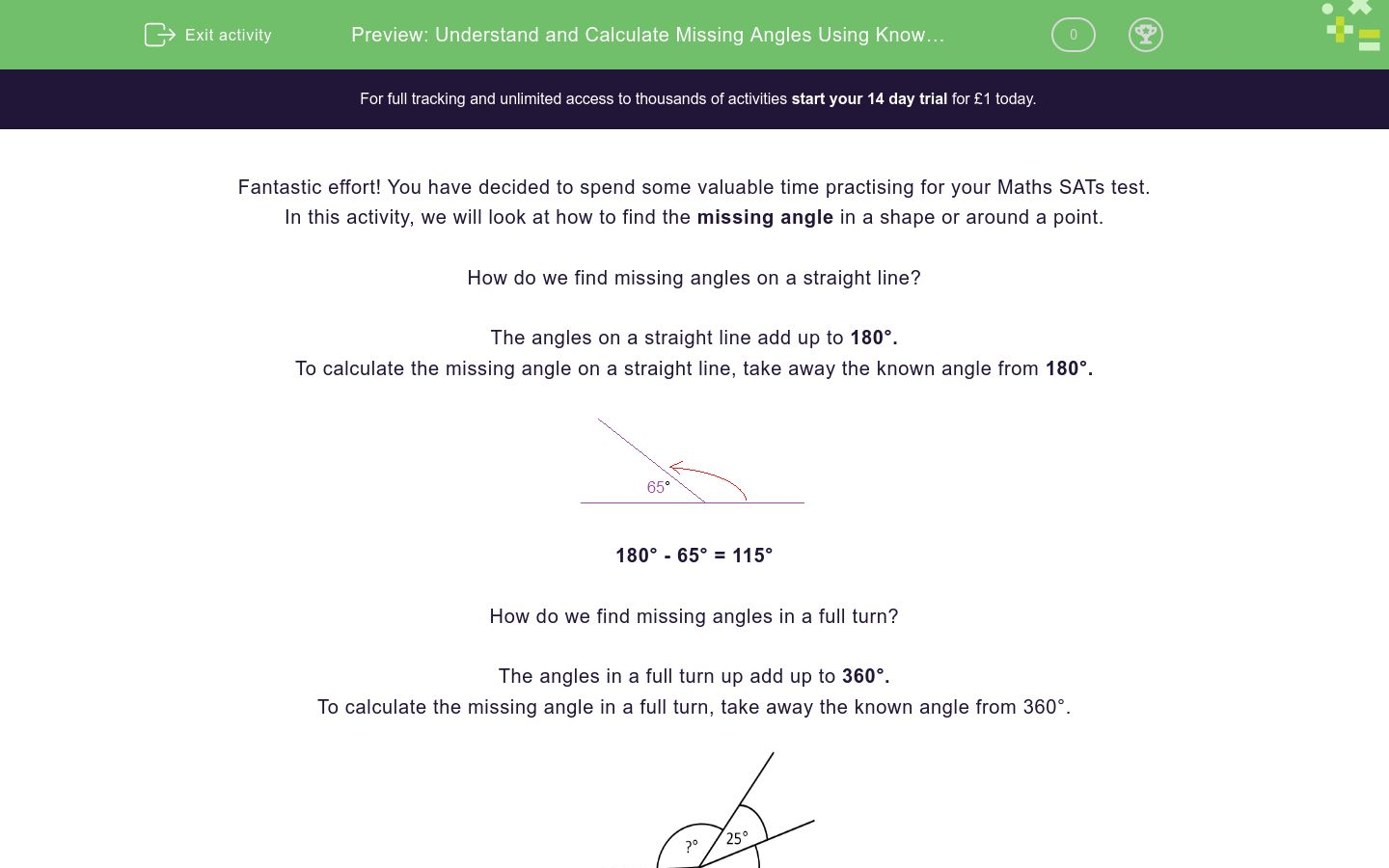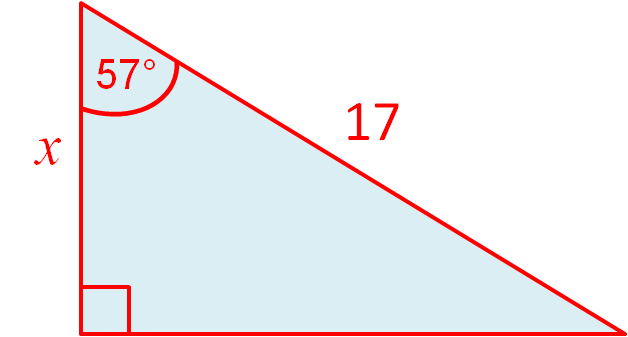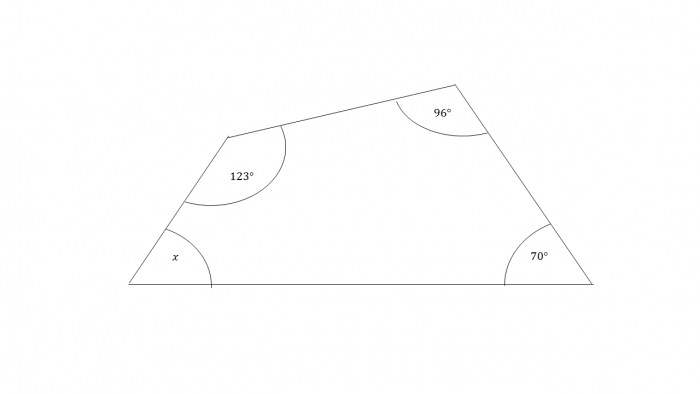# Understand and Calculate Missing Angles Using Known Angle Facts

In this worksheet, students will be asked to find the missing angles in a shape or around a point using known angle facts.Key stage:  KS 2

Curriculum topic:   Exam-style Questions: SATs Maths

Curriculum subtopic:   Exam-Style Questions: Using Known Facts

Difficulty level:### QUESTION 1 of 10

Fantastic effort! You have decided to spend some valuable time practising for your Maths SATs test.

In this activity, we will look at how to find the missing angle in a shape or around a point.

How do we find missing angles on a straight line?

The angles on a straight line add up to 180°.

To calculate the missing angle on a straight line, take away the known angle from 180°.180° - 65° = 115°

How do we find missing angles in a full turn?

The angles in a full turn up add up to 360°.

To calculate the missing angle in a full turn, take away the known angle from 360°.105° + 90° + 25° = 220°

360° - 220° = 140°

How do we find missing angles on intersecting lines?

All 4 angles will add up to 360°.

Opposite angles on a cross are equal.Step 1 (we know the angle opposite 65° is also worth 65° so add these together): 65° + 65° =130°

Step 2 (subtract the total of these 2 angles from 360°): 360° - 130° = 230°.

Step 3 (Divide this total by 2 to find what each of the 2 remaining angles is worth): 230°÷ 2 =115°

How do we find missing angles in a triangle?

The angles in a triangle always add up to 180°.

To find the missing angle, add together the known angles and subtract from 180°.If you are given a triangle like this, but asked to find the missing angle, focus only on the angles given, ignore the other information.

57° + 90° = 147°

180° - 147° = 33°

How do we find missing angles in a quadrilateral?

A quadrilateral is a shape with 4 straight sides, like a square, rhombus, parallelogram, trapezium, rectangle.

To find the missing angle, add together the known angles and then subtract from 360°90° + 90° + 40° = 220°

360° - 220° = 140

Now we have revised those key facts, time to have a go at some practice questions!What is the missing angle worth on this straight line?

120°

90°

95°

100°

What is the missing angle in this triangle worth?120°

90°

95°

100°

What is angle X worth around this point?120°

90°

95°

100°

Can you match the statements with the correct label?## Column B

Angles on a straight line =
360°
Angles around a point =
180°
Angles in a triangle =
360°
180°
Angles on intersecting lines =
360°

Here are some intersecting lines.

Can you correctly match the value of angles a, b and c?## Column B

a =
55°
b =
125°
c =
125°

What is angle b worth on these intersecting lines?## Column B

a =
55°
b =
125°
c =
125°

What are angles a and c worth on these intersecting lines?## Column B

a =
55°
b =
125°
c =
125°

What is angle x worth in this quadrilateral?## Column B

a =
55°
b =
125°
c =
125°

What is the missing angle on the straight line worth?## Column B

a =
55°
b =
125°
c =
125°

Can you calculate angle c?## Column B

a =
55°
b =
125°
c =
125°
• Question 1What is the missing angle worth on this straight line?

90°
EDDIE SAYS
How did you do on this first question? Did you spot that the missing angle must be worth 90° as the given angle is worth 90°? 180°- 90° = 90°. Remember that 90° is the same as a right angle.
• Question 2

What is the missing angle in this triangle worth?EDDIE SAYS
Don't worry if you found that tricky, the more you practice, the easier it gets! 90° + 35° = 125° 180° - 125° = 55° Remember the angles in a triangle will always add up to 180°.
• Question 3

What is angle X worth around this point?EDDIE SAYS
Great effort! There were 2 stages to complete to find that the missing angle X is worth 102°. Remember that the angles around a point will always add up to 360° 118° + 90° + 50° = 258° 360° - 258° = 102°
• Question 4

Can you match the statements with the correct label?## Column B

Angles on a straight line =
180°
Angles around a point =
360°
Angles in a triangle =
180°
360°
Angles on intersecting lines =
360°
EDDIE SAYS
Did you get the hang of this one? There was a lot to think about and match but once you know these rules, it really does make finding missing angles so much easier! Keep going- you are making super progress!
• Question 5

Here are some intersecting lines.

Can you correctly match the value of angles a, b and c?## Column B

a =
125°
b =
55°
c =
125°
EDDIE SAYS
Phew, that was a toughie! When working out the angles on intersecting lines, remember that opposite angles are equal. So, straight away, we know that angle b = 55° Next, we add 55° + 55° = 110° Subtract this from the total of 360° 360° - 110° = 250° Finally, divide 250° by 2 to find what the last 2 angles are worth. 250 ÷ 2 = 125° You are now halfway through this activity, keep going!
• Question 6

What is angle b worth on these intersecting lines?EDDIE SAYS
How are you doing? On these intersecting lines, angle b is worth 27°. We don't need to do any working out for this question as we know that opposite angles are equal on intersecting lines.
• Question 7

What are angles a and c worth on these intersecting lines?EDDIE SAYS
Nice job if you wrote 153°. To solve this one, we had to carry out the 3 steps of working out. Step 1 = 27° + 27°= 54° Step 2 = 360° - 54° = 306° Step 3 = 306° ÷ 2 = 153° You're making super progress- keep going now!
• Question 8

What is angle x worth in this quadrilateral?EDDIE SAYS
Take a deep breath, you've got this! The angles in a quadrilateral always add up to 360°. Use the angles we have, add them together and then subtract from 360°. 123° + 96° + 70° = 289° 360° - 289° = 71°
• Question 9

What is the missing angle on the straight line worth?EDDIE SAYS
This is a tricky one! Great work if you spotted that the missing angle is 32°. To find the answer we had to firstly add together the angles we had: 78° + 70° = 148° 180° - 148° = 32°
• Question 10

Can you calculate angle c?EDDIE SAYS
Phew, that was a challenge! Great job if you wrote 40°. To find the angle c, we had to remember that vertically opposite angles are equal. So, we had to subtract 17° from 57° = 40° Well done! That is another activity completed, keep up the great work!
---- OR ----

Sign up for a £1 trial so you can track and measure your child's progress on this activity.

### What is EdPlace?

We're your National Curriculum aligned online education content provider helping each child succeed in English, maths and science from year 1 to GCSE. With an EdPlace account you’ll be able to track and measure progress, helping each child achieve their best. We build confidence and attainment by personalising each child’s learning at a level that suits them.

Get started